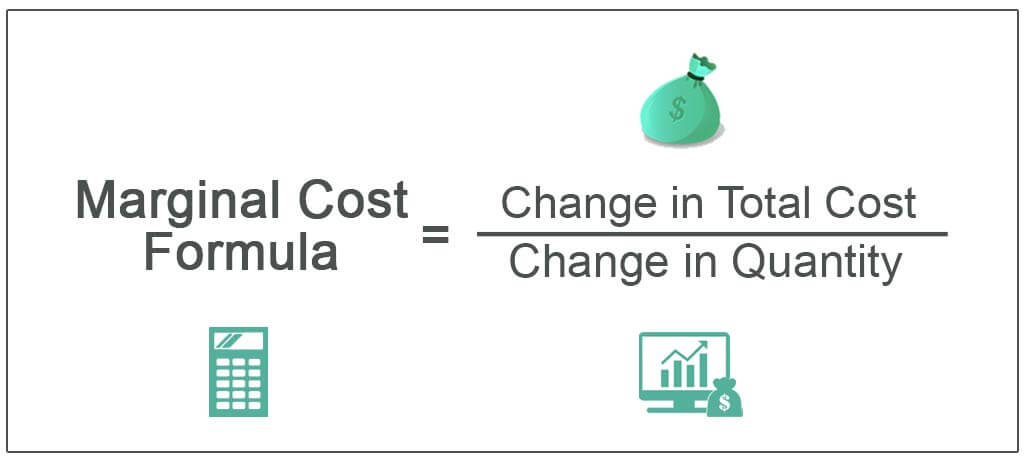Marginal cost: Definition, formulas, curves and more

Marginal cost definition With the increase in one unit of output, the total cost increases, and the total increased unit is the marginal cost. Moreover, marginal cost is a change in total cost by producing an additional unit of output. Suppose we produce five units…